### Home > CAAC > Chapter 13 > Lesson 13.OF2-S > Problem7-138

7-138.
1.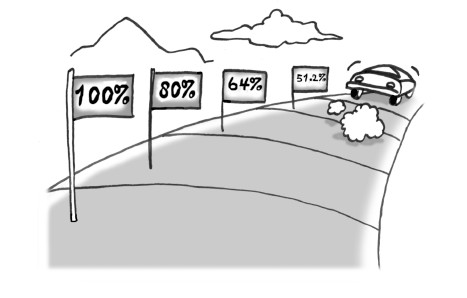A rule-of-thumb used by car dealers is that the trade-in value of a car decreases by 20% of its value each year. Homework Help ✎

1. Explain how the phrase “decreases by 20% of its value each year” tells you that the trade-in value varies exponentially with time (i.e., can be represented by an exponential function).

2. Suppose the initial value of your car is $23,500. Write an equation expressing the trade-in value of your car as a function of the number of years from now. 3. How much will your car be worth in four years? 4. In how many years will the trade-in value of your car be$6000?

5. If your car is really 2.7 years old now, what was its trade-in value when it was new?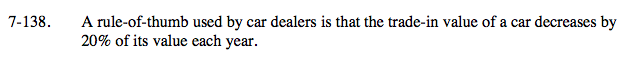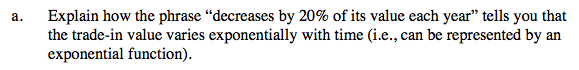'Decreases by 20%' means that you multiply by 0.8 each year. A sequence with a multiplier is geometric and has an exponential rule.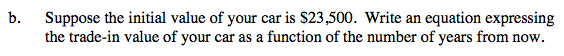y = 23500(0.8)x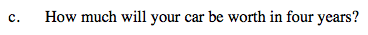Use the equation that you found in part (b).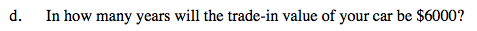6000 = 23500(0.8)x

Divide by 23,500 first then take the log of both sides.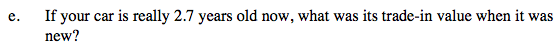You are solving for A, which is 23,500 in part (b). The current price will be $23,500, and x will be 2.7. Your answer should be larger than$23,500.